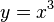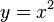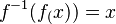# One-one function

A function$f$ is called one-one function if it never adopts the same value twice. This means, one-one functions return a unique range for each element of their domain.

Other names given to the one-one function are one to one, or injective function.

One-one functions are a set of functions which denote the relation between sets, elements or identities. The other two sets are surjective functions and bijective functions.

## Definition

The function$f(x)$ is called one-one function when for every value of$x$ in the domain of the function, there will be a unique value of$f(x)$.

## Geometric proof

Similar to the vertical line test (VLT) for functions, there is a horizontal line test (HLT) to prove the one-one property. A function is one-one if and only if no horizontal line intersects its graph more than once.

In the graph below, the function$y=x^3$ is intersected once by the horizontal line. Therefore the function is geometrically proven to be one-one.

In the graph below, the function$y=x^2$ is intersected twice by the horizontal line. Therefore the function is geometrically proven no to be one-one.

## Properties

• The domain of f equals the range of f-1.
•$f^{-1}(f_(x))=x$ for every x in the domain of f and f
• The graph of a function and the graph of its inverse are symmetric with respect to the line y=x.
• If f and g are both one-one, then f°g follows injectivity.
• If g°f is one-one, then function f is one-one, but function g may not be.
• A one-one function is either strictly decreasing or strictly increasing.
• A function that is not a one-one is considered as many-to-one.
• Parabolic functions are not one-one functions.

## Examples

Examples of one-one functions include:

• Identity function: f(x) is always injective.